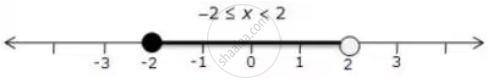Share

X ∈ {Real Numbers} and -1 < 3 – 2x ≤ 7, Evaluate X and Represent It on a Number Line. - ICSE Class 10 - Mathematics

ConceptLinear Inequations in One Unknown

Question

x ∈ {real numbers} and -1 < 3 – 2x ≤ 7, evaluate x and represent it on a number line.

Solution

-1 < 3 - 2x <= 7

-1 < 3 - 2x  and 3 - 2x <= 7

2x < 4 and 2x <= 4

x < 2 and x >= -3

Solution set = {-2 <= x < 2, x ∈ R}

Thus, the solution can be represented on a number line as:Is there an error in this question or solution?

APPEARS IN

Solution X ∈ {Real Numbers} and -1 < 3 – 2x ≤ 7, Evaluate X and Represent It on a Number Line. Concept: Linear Inequations in One Unknown.
S